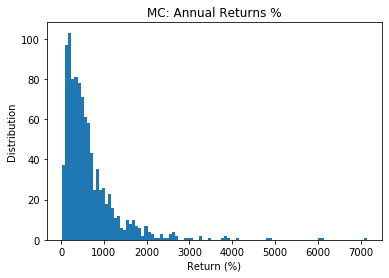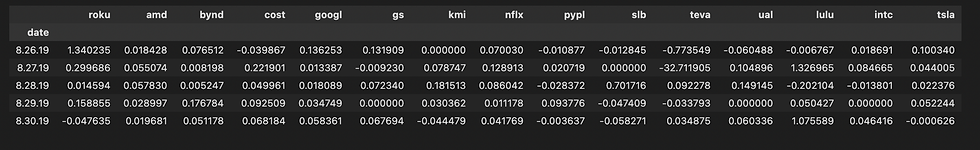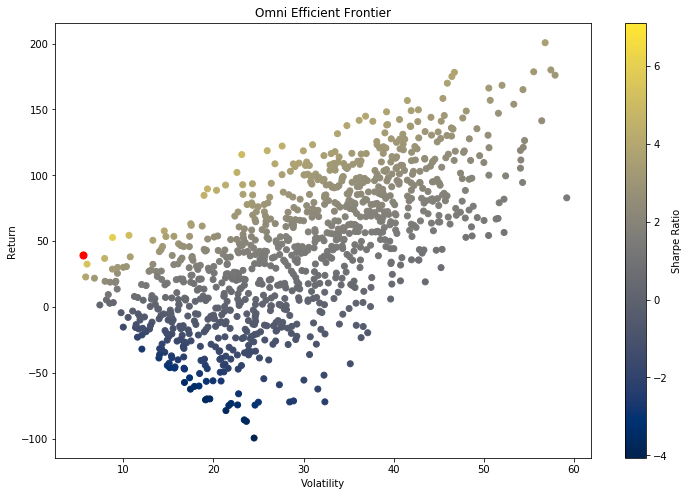top of page

# Monte Carlo Simulation

• A Monte Carlo simulation is a model used to predict the probability of different outcomes when the intervention of random variables is present.

• Monte Carlo simulations help to explain the impact of risk and uncertainty in prediction and forecasting models.

• A variety of fields utilize Monte Carlo simulations, including finance, engineering, supply chain, and science.

• The basis of a Monte Carlo simulation involves assigning multiple values to an uncertain variable to achieve multiple results and then averaging the results to obtain an estimate.

• Monte Carlo simulations assume perfectly efficient markets.

If you need to solve a problem that you cannot ﬁgure out, chances are Monte Carlo simulations can be used to get you pretty close to correct. Recently I was trying to ﬁnd a way to optimize a complex strategy that utilizes spreads of spreads in Futures markets, and this optimization algorithm was killing me. I decided that I could set the spread ratio as a random variable, and run it as a Monte Carlo simulation, and at least get in the right direction — in 5 minutes & 100k iterations I had a simple 15-line solution to a problem that had taken me maybe 350 lines of Python when I initially tried to use a minimization function. This is the adjustable wrench of your toolbox.

Let’s dive in, and I’m going to over-comment this code so it could not be clearer what’s doing what. First off, we need your security returns. This can be pulled from system performance (Like my example) or pulled with quantrautil using simple price data. The DF should be Securities Tickers as columns, with date rows and daily return values. Technically, it should be log returns so I’ve included that calculation — but the difference is typically immaterial.

Begin by initializing the arrays for saving the performance values from the Monte Carlo runs, and set up the Monte Carlo simulations loop, deﬁning the # of runs as high as your PC will allow (start with 1k or so, scale up).

The important thing to remember is the weights, this is the Monte Carlo simulations magic, as this will select a new value each time and provide the power to the optimization, making each run unique. Also notice how each value your saving (ret, vol, Sharpe) is really an array being saved with indexing per each run — so run 0 begins, randomizes weights, and calculates the return, saving it to index 0 in ret arr, and then vol saves in 0 index, and ﬁnally SR. Once you’ve looped through, you run an argmax() function in the SR arr (or whatever value you maximizing), which will give you weight, 477 in my example. This mean run 477 gave you the highest SR value, and that is your ideal weight! You can ﬁnd the optimal values in all weights, indexing with your max run number.

(I also included a helper function to save the weights and tickers into a DF, and Pkl it for reference).

```import numpy as np
import numpy.random as nrand
import matplotlib.pyplot as plt
import math

#Define vars
S = 1000000
T = 252
mu = 1.92246
vol = .86123  #Between .76 and .86

result = []
for i in range(1000):
daily_returns = np.random.normal(mu/T,vol/math.sqrt(T),T)+1

price_list = [S]

for x in daily_returns:
price_list.append(price_list[-1]*x)
result.append(price_list[-1]) #appending each runs end value --to calculate the mean return

plt.plot(price_list)#This is key, KEEP THIS IN LOOP,votherwise it will plot one iteration/return path.
plt.title('Daily MC')
plt.show()``````from scipy import stats
from statistics import stdev

print('Mean:',np.mean(result))
print('Mean Ret:',np.mean(result)/S*100)
print('Median:',np.median(result))
print('Median Ret:',np.median(result)/S*100)
print('Min:',np.min(result))
print('Min Ret:',np.min(result)/S*100)
print('Max:',np.max(result))
print('Max Ret:',np.max(result)/S*100)
#print('Mode:',stats.mode(result))
print('Stdev', stdev(result))
mc_mu = np.mean(result)
med = np.median(result)
mc_min = np.min(result)
mx = np.max(result)
std = stdev(result)

metrics = [mc_mu,med,mc_min,mx]
print('sharpe:',mu/vol)

print('5% Quantile',np.percentile(result,5))
print('5% Quantile %',np.percentile(result,5)/S*100)
print('95% Quantile',np.percentile(result,95))
print('95% Quantile %',np.percentile(result,95)/S*100)``````'''Histogram (A much more usable plot!)'''

res = [i/S*100 for i in result]
plt.title('MC: Annual Returns %')
plt.xlabel('Return (%)')
plt.ylabel('Distribution')
plt.hist(res,bins=100)
plt.show()

print('Mean return %:',np.mean(res))
print('Median return %:',np.median(res))
print('Min return %:',np.min(res))
print('Max return %:',np.max(res))
#print('Mode:',stats.mode(result))
print('Stdev %', stdev(res))

``````import pandas as pd

'''Hint -- if youre having issues with path -- drag and drop file into terminal, copy abs path!'''

o = omni.iloc[1:23,0:16]

o.columns = ['date','roku','amd','bynd','cost','googl','gs','kmi','nflx','pypl','slb','teva','ual','lulu','intc','tsla']
#omni.iloc

'''Fix the sheet! (sorry -- practicing bad data science of more than 1 value per cell : / )'''
o.iloc
o['date'].iloc = '8.28.19' #Fix the asterisk
o.iloc

pct = o.set_index('date').cumsum(axis=0).pct_change().fillna(0)
pct.tail()``````'''Monte carlo method -- efficient frontier'''

#Monte Carlo -- Portfolio Optimization
#np.random.seed(100)

##INCLUDE DATA HERE

#Normalize returns--Nat Log -- Technically, it uses log returns, but the difference is immaterial.
#log_ret = np.log(ret/ret.shift(1)) #Calc for log returns, if official is important (This is instead of pct_change())
log_ret = pct
ret = log_ret
#Create Temporary (Random) weights
weights = np.array(np.random.random(15)) #USE NUM SECURITIESS

#Rebalance w/ constraints (CANNOT BE > 1)
weights = weights/np.sum(weights)
print(weights)

#DEFINE ARRAYS FOR STORING METRICS
num_runs = 1000    #Kick this number up 10k-100k for better results
all_weights = np.zeros((num_runs,len(ret.columns)))
ret_arr = np.zeros(num_runs)
vol_arr = np.zeros(num_runs)
sharpe_arr = np.zeros(num_runs)

#Begin MC Loop
for run in range(num_runs):

#Weights
weights = np.array(np.random.random(15))  #CHG to number securities *** THIS is the key to MC, this random value creation for weights
#If you're looking for a challenge, try using a poisson, gamma or Student T dist!
weights = weights/np.sum(weights)

#Save weights (For reference Later)
all_weights[run,:] = weights

#Expected Ret (Record each runs return in ret_arr)
exp_ret = np.sum((log_ret.mean() * weights) * 252)
ret_arr[run] = np.sum( (log_ret.mean() * weights) * 252) #Time =  year

#Exp Vol: (Lets attempt some linear algebra w/out runtime error!)
exp_vol = np.sum((log_ret.std() * weights) * 252)
# Sqrt of dot product of Transposed weights X Cov of Log returns & weights--whew.
vol_arr[run] = np.sqrt(np.dot(weights.T,np.dot(log_ret.cov()*252,weights)))

#Sharpe
SR = exp_ret/exp_vol
sharpe_arr[run] = ret_arr[run]/vol_arr[run]``````#sharpe_arr.argmax()
#MC_SR = sharpe_arr #Plug in to sharpe_arr10.094382

#find max SR per vol arr and SR array (for plotting)
max_sr_vol = vol_arr[sharpe_arr.argmax()]
max_sr_ret = ret_arr[sharpe_arr.argmax()] #85563 is max idx

#Save optimal weights, calc SR_annualized,
idx = sharpe_arr.argmax()
#all_weights[idx]
MC_SR = sharpe_arr[idx]
print(MC_SR)
SR_ann = np.sqrt((MC_SR))*12
SR_ann

print('Max Sharpe:',MC_SR)
print('Max Annualized Sharpe',SR_ann)
print('max sr ret:',max_sr_ret)
#print('Max sr vol:',max_sr_vol)``````import matplotlib.pyplot as plt
'''Plot the Markowitz efficient frontier'''
plt.figure(figsize=(12,8))
plt.scatter(vol_arr, ret_arr, c=sharpe_arr, cmap='cividis')
plt.colorbar(label='Sharpe Ratio')
plt.xlabel('Volatility')
plt.ylabel('Return')
plt.title('Omni Efficient Frontier')
plt.scatter(max_sr_vol, max_sr_ret,c='red', s=50) # red dot
plt.show()``````'''
-- Helper functions --
Once you have the optimal weights (list), you can pull them up in a DF with their tickers (rather than trying to match with order)
AND you can pkl the ^^ weights df for later reference!
(nothing worse than running a 500k MC to forget to run them, or accidentally start it again)'''

def create_wts_df(min_func,sec_df):
'''Creates df of optimization result weights w/ respective tickers'''
data = {'Sec':sec_df.columns,'Wt':all_weights[idx]}
res = pd.DataFrame(data).sort_values(by='Wt',ascending=False).set_index('Sec')
return res

def create_pkl(df,filename):
'''Pkls the weights, so you can save them for later!'''
name = filename + '.pkl'
df.to_pickle(name)
return '{} file pickled'.format(name)

#res.to_pickle('TRIPLE_opt_flr.pkl') #Simple pkl```
``` '''Little extra fun -- correlation!'''

corr = pct.corr()
import seaborn as sns
ax = sns.heatmap(corr)
ax.figure.set_size_inches(14,10)````#-Happy Trading ! 🤠 😊`

See All
bottom of page